# 978-0123869449 Chapter 12 Part 1

Document Type
Homework Help
Book Title
Authors
Michael F. Modest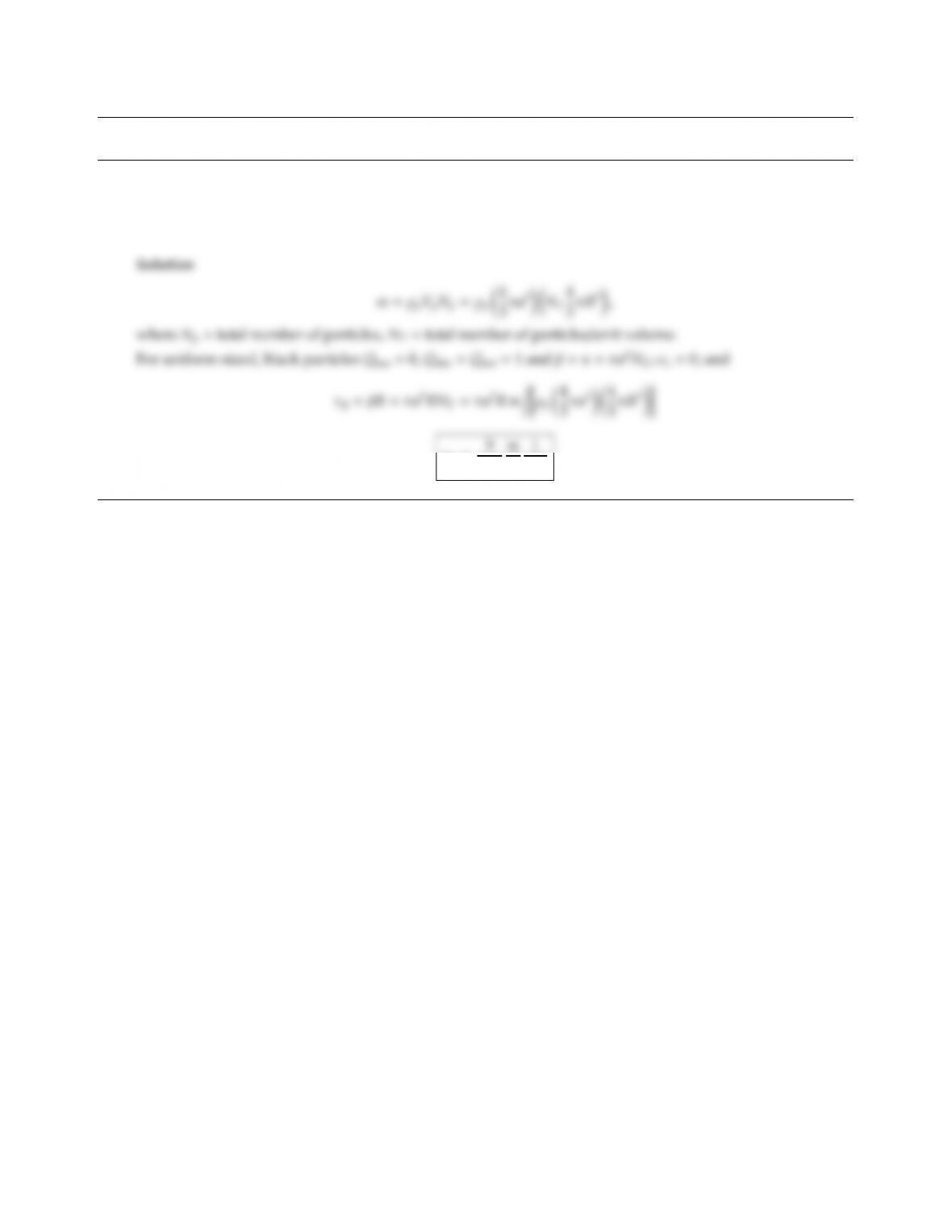CHAPTER 12
12.1 A mass of m(kg) of coal is ground into particles of equal size a(µm), which may be assumed to be “large” and
black. Determine the optical thickness of the resulting spherical particle cloud, assuming that the particles
are uniformly distributed throughout the volume of a sphere of radius R.
τR=9
16π
ρp
aR2
339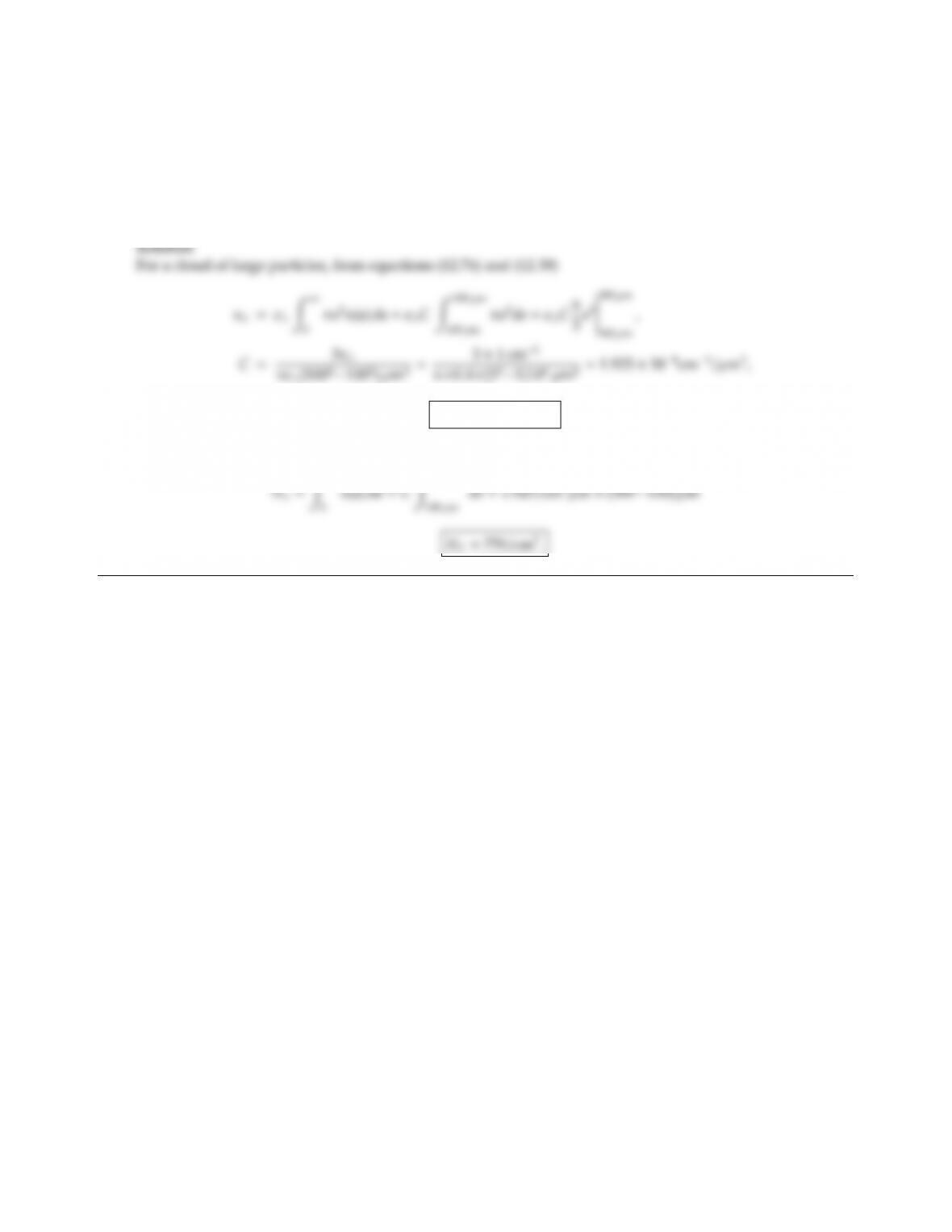12.2 One way to determine the number of particles in a gas is to measure the absorption coecient for the cloud.
For a cloud of large, diuse particles (x1, ǫλ=0.4), the particle distribution function is known to be of the
form
n(a)=(C=const,100 µm<a<500 µm,
0,elsewhere.
If κλis measured as 1 cm1, determine Cand the total number of particles per cm3.
C=1.925/cm3µm.
The total number of particles is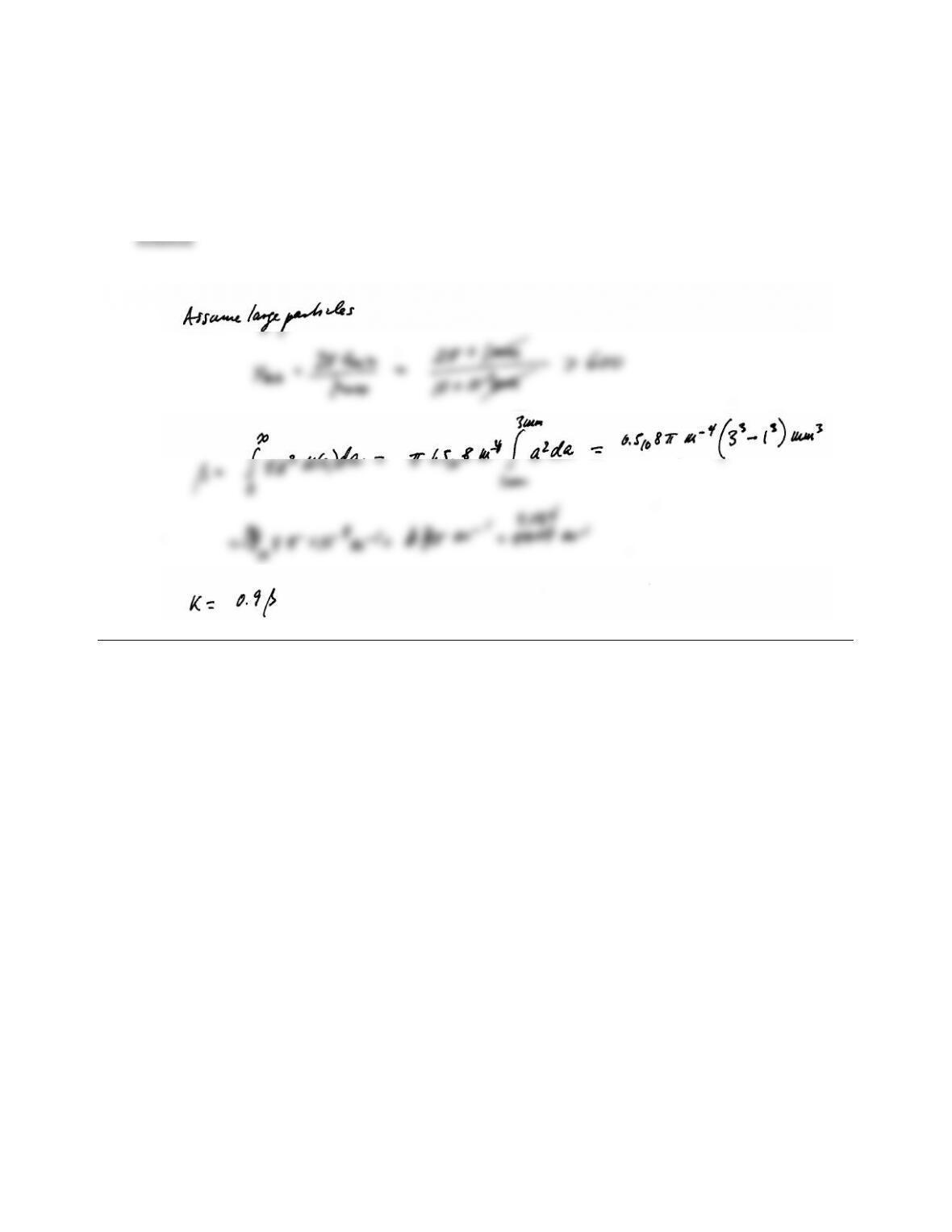CHAPTER 12 341
12.3 Coal particles (gray and diuse with ǫ=0.9, m=1.925 0.1i) are burnt in a long cylindrical combustion
chamber. The combustor is well stirred, resulting in a uniform distribution of particles with a size distribution
of
n(a)=1.5×108m4,amin =1 mm <a<amax =3 mm,
0,otherwise.
Determine absorption and scattering coecient of this particle cloud.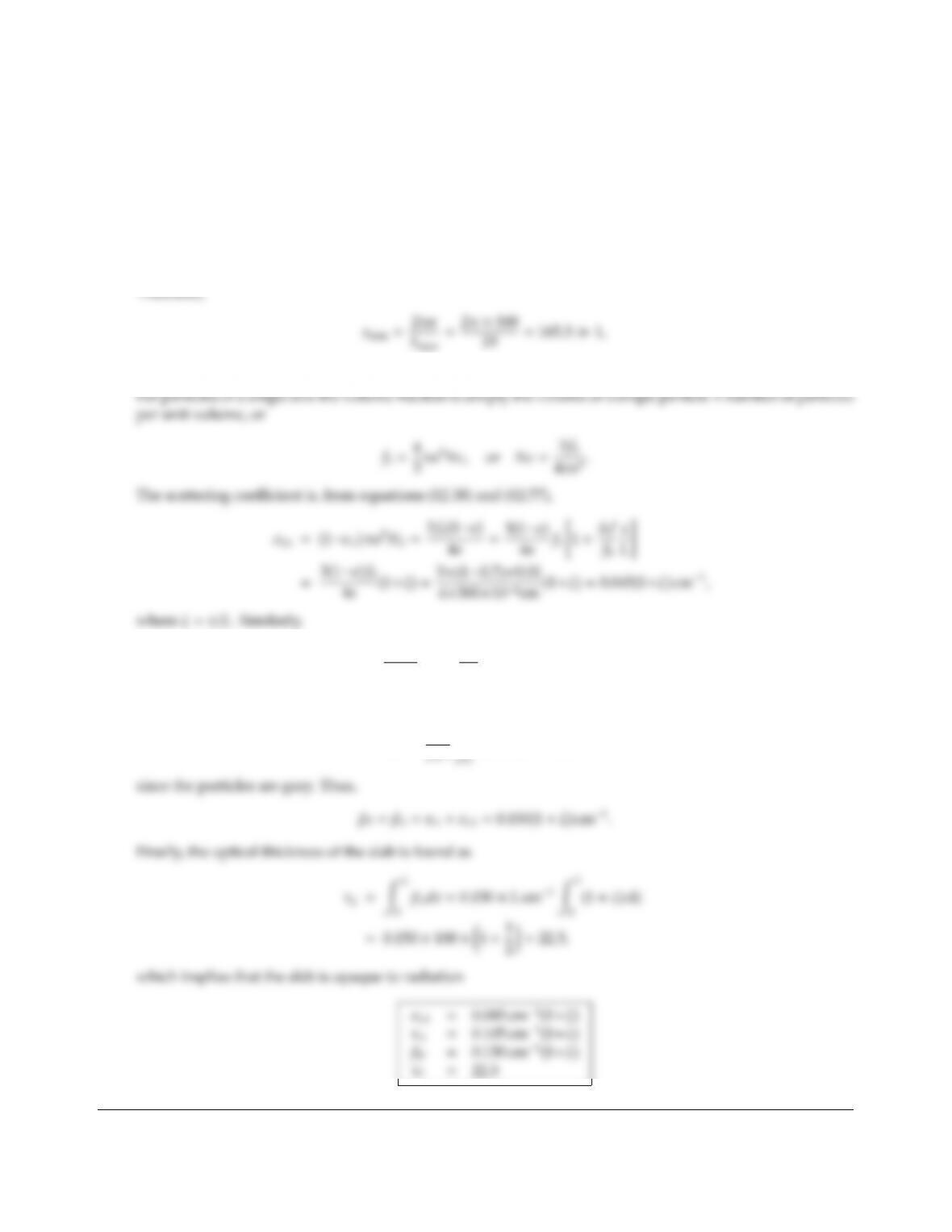12.4 Consider a particle cloud of ﬁxed-size particles (radius a) contained between parallel plates 0 xL=1 m.
The volume fraction of particles is fv(x)=f0+ f(x/L), and their temperature is T(x)=T0+ ∆T(x/L), where
f/f0= ∆T/T0=1, f0=1%, T0=500 K. Assuming the particle size to be a=500 µm, and made of a
material with a gray hemispherical emittance of ǫλ=0.7, show that the large-particle approximation may be
used for the infrared. Calculate the local, spectral absorption and scattering coecients. Determine the local
Planck-mean extinction coecient as well as the total optical thickness of the slab (based on the Planck-mean).
Solution
With temperatures ranging between 500 K and 1000 K, the Planck function Ibλis substantial in the wavelength
range 2.2µm< λ < 19 µm (i.e., the range between f(λT)min 0.1 and f(λT)max 0.9 from Appendix C).
so that the large particle limit applies.
κλ=ǫλπa2NT=ǫλ
1ǫλ
σsλ=0.7
0.3×0.045(1+ξ)=0.105(1+ξ) cm1.
The local Planck-mean extinction coecient is found from equation (12.32) as
βP=π
βλIbλdλ=βλ,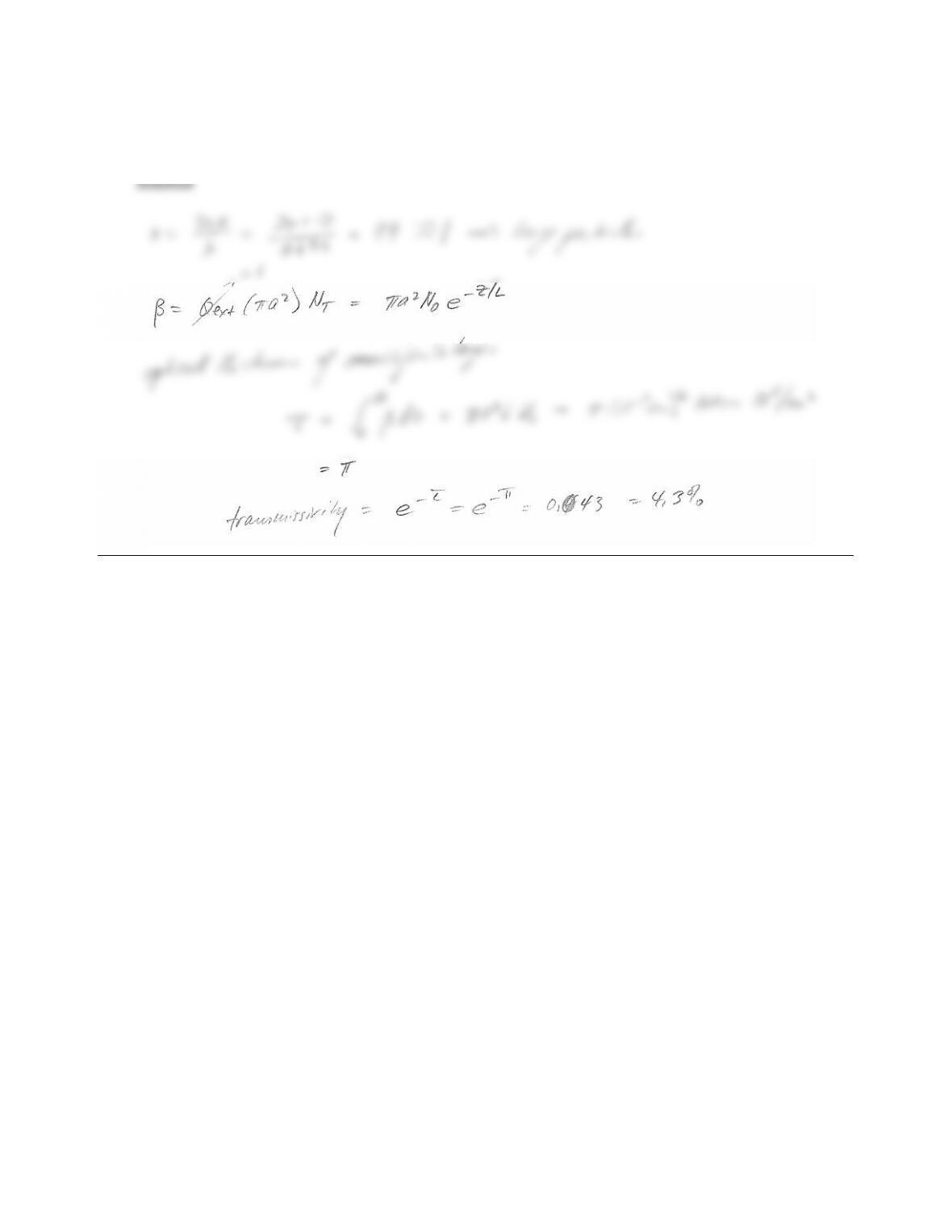CHAPTER 12 343
12.5 Black spheres of radius a=10µm occupy a semiinﬁnite space with varying number density NT=N0ez/L
(N0=104/cm3,L=1 m). If a HeNe laser (λ=0.633µm) shines onto this layer (at z=0 into z-direction), what
fraction of its energy is directly transmitted?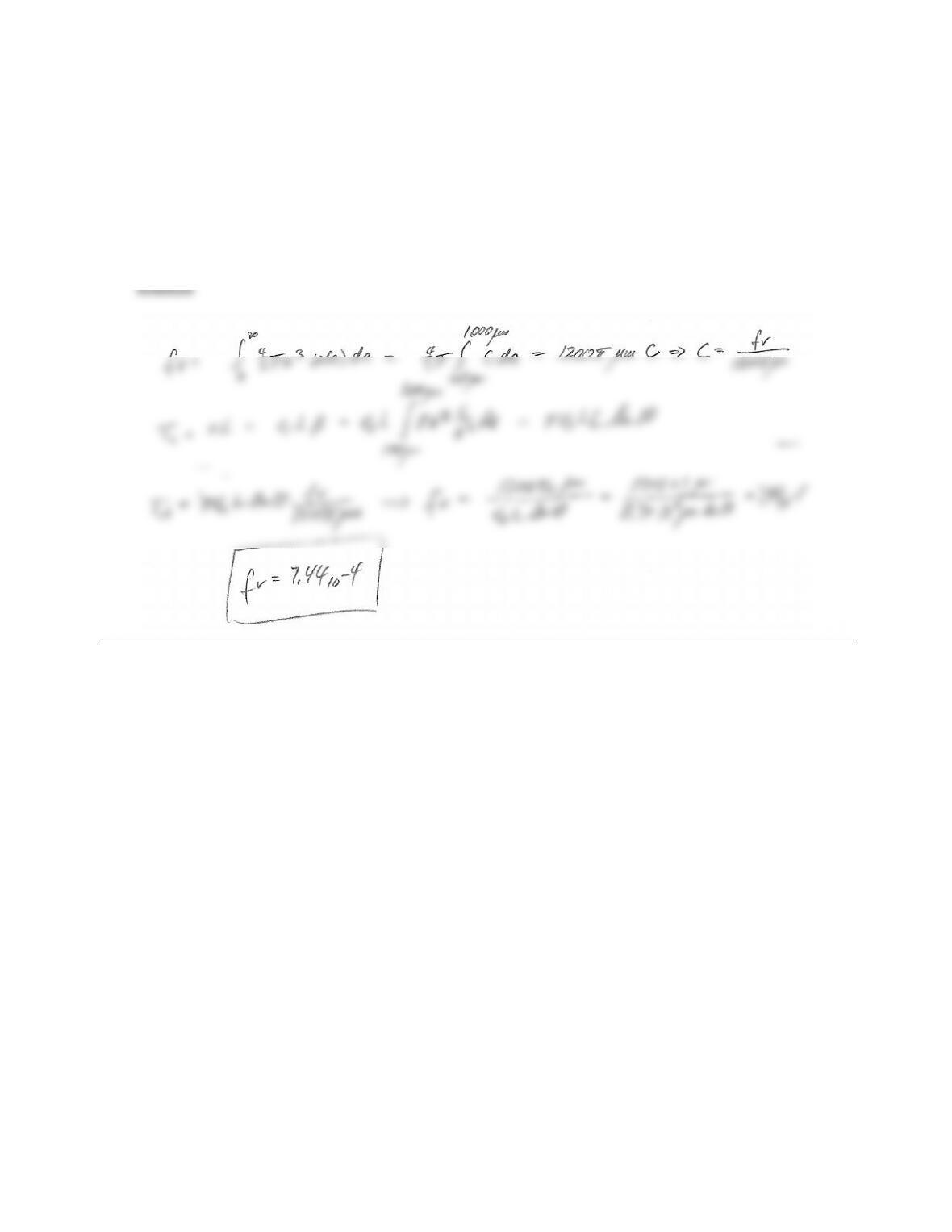12.6 Pulverized coal is burned in a combustor. In order to achieve maximum radiative heat transfer rates, it is
desired to keep the optical thickness of the particle cloud at intermediate levels, say τL=κL=1, in the
intermediate infrared, or λ=5µm, where L=1 m is a characteristic combustor dimension. Determine the
necessary volume fraction of coal dust, if its size distribution may be taken as
n(a)(a3,100 µma1000 µm,
0,all other a.
The spectral, hemispherical emittance of coal at λ=5µm is ǫλ=0.7.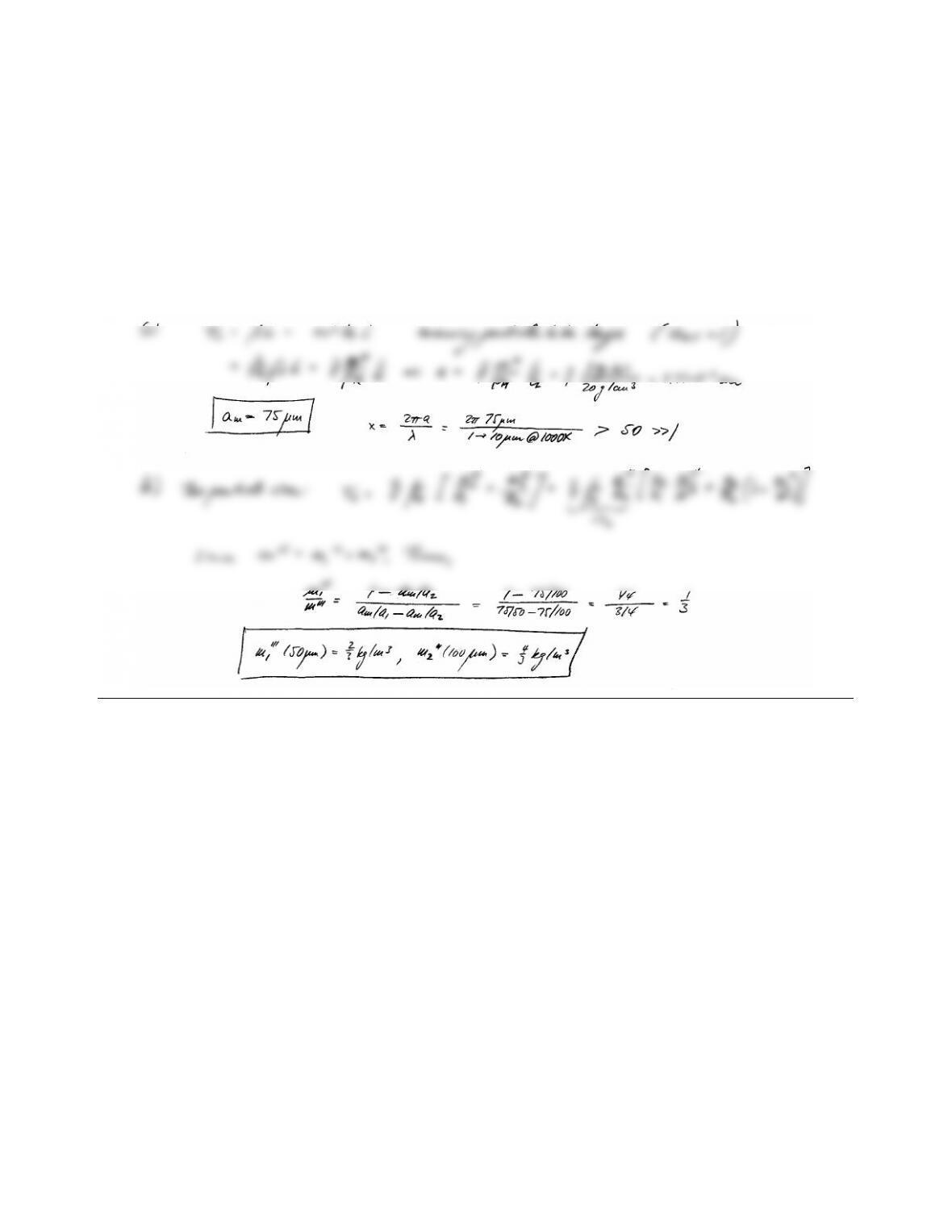CHAPTER 12 345
12.7 To maximize radiative heat loss from a hot medium it is usually desirable for the medium to have an
intermediate optical thickness. A nonparticipating hot gas at 1000 K occupying the 1 m wide space between
two parallel plates is to be seeded with platinum particles to make the optical thickness of the slab (based on
extinction) unity (τL=1.0). For this purpose a total of 2 kg/m3particles are to be used (density of platinum
20 g/cm3).
(a) To what particle radius must the platinum be ground in order to achieve the desired result?
(b) Instead, if platinum spheres of radii 50 µm and 100 µm are available, how much of each (for a total of
2 kg/m3) must be used to achieve the same result?
Solution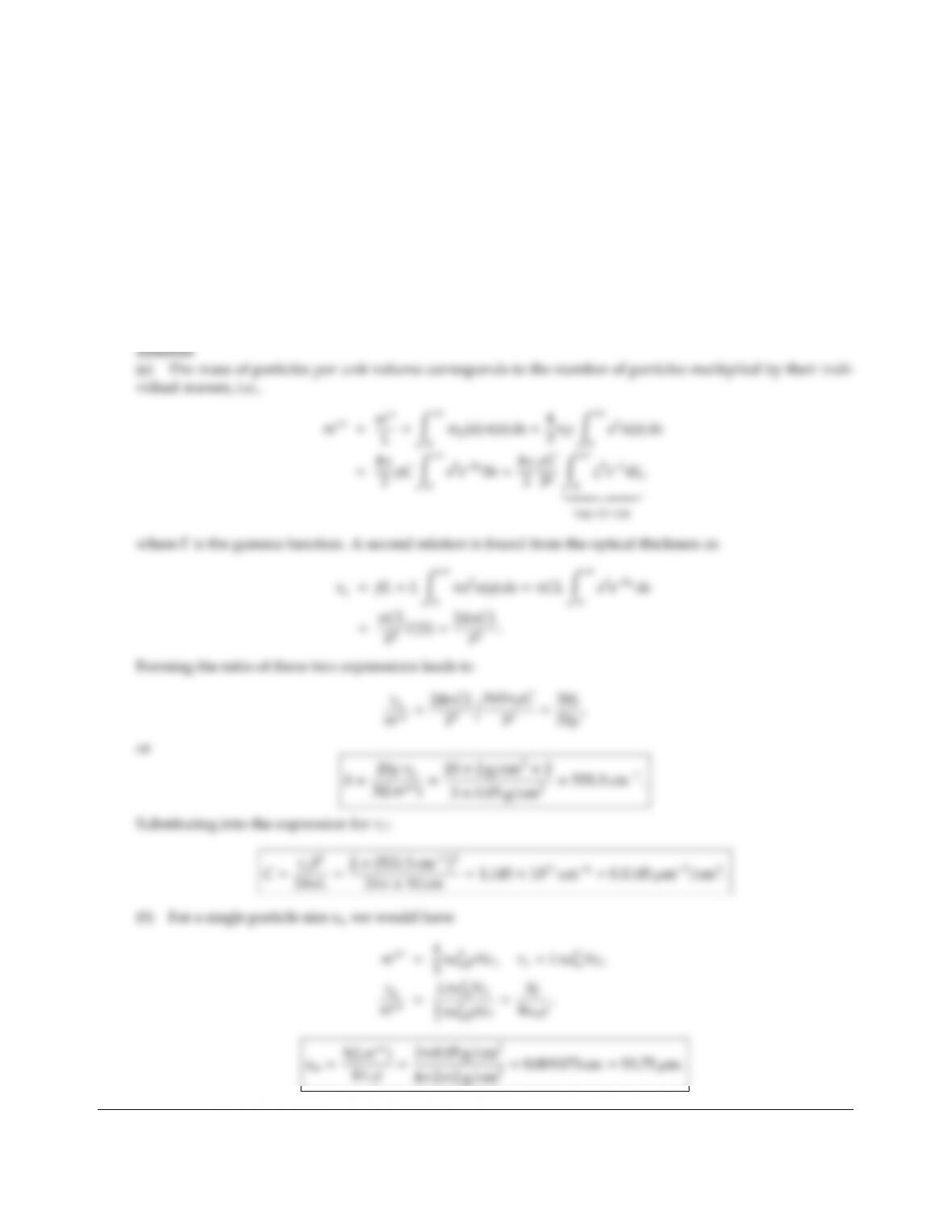12.8 The distribution function of a particle cloud may be approximated by an exponential function such as
n(a)=Ca2eba, where ais particle radius and band Care constants. It is proposed to determine the distribution
function of a set of particles by suspending a measured mass of particles between parallel plates, followed by
measuring extinction across the particle layer. Given that m′′ =0.05 g/cm2of particles are present between
the plates, which are 10 cm apart, and that the optical thickness based on extinction has been measured as
τ0=2,
(a) Determine the distribution function above (i.e., band C).
(b) If a single particle size were to be used to achieve the same extinction with the same mass of particles,
what would the particle radius be?
You may assume all particles to be “large” and diuse spheres with an emittance of 0.7 and a density of
ρ=2 g/cm3.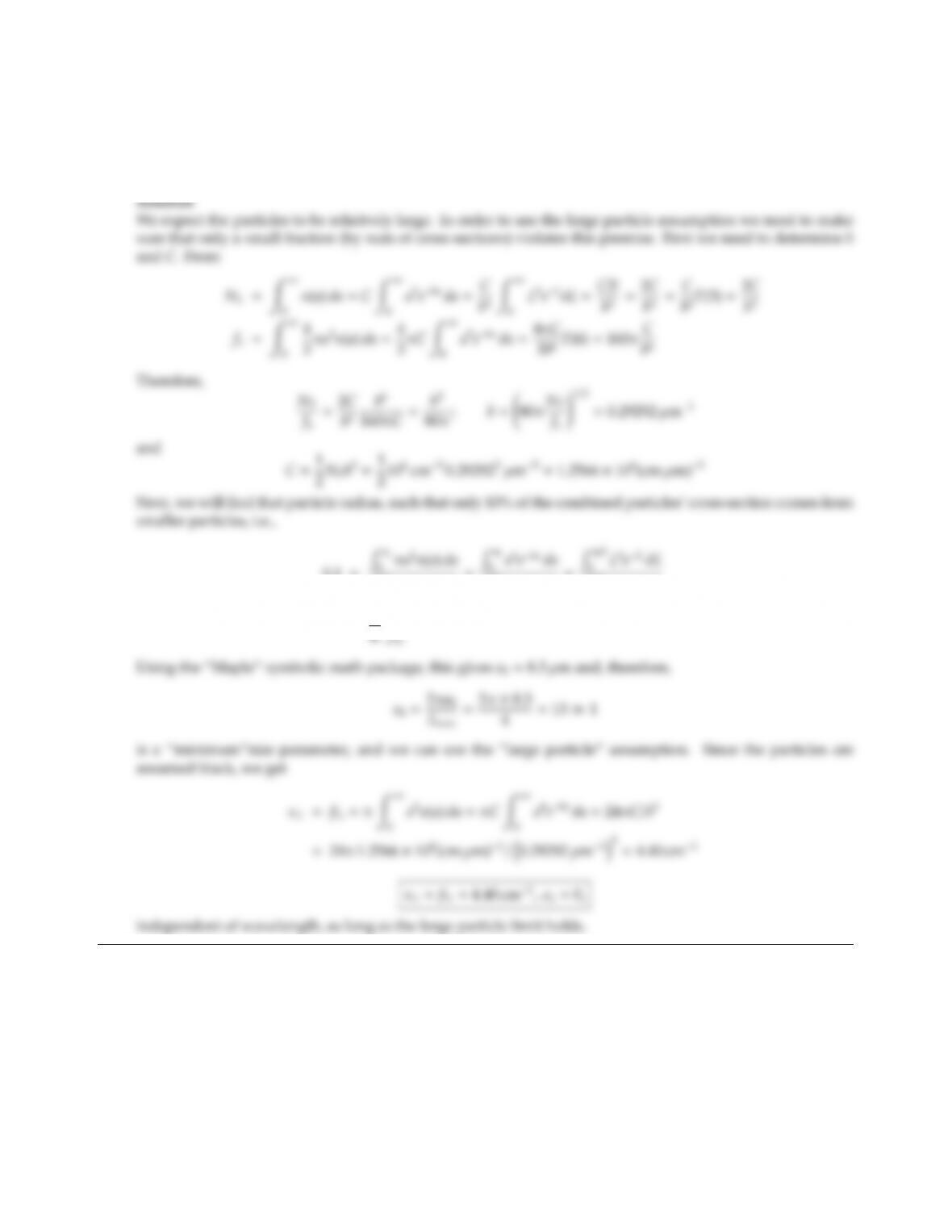CHAPTER 12 347
12.9 Consider a particle cloud with a distribution function of n(a)=Ca2eba, where ais particle radius and band C
are constants. The particles are coal (ǫ=1), and measurements show the particles occupy a volume fraction
of 1%, while the number density has been measured as NT=106/cm3. Calculate the extinction, absorption,
and scattering coecients of the cloud for the wavelength range 1 µm<λ<4µm.
0.1=Ra0
R
0πa2n(a)da
=Ra0
R
0a4eba da
=Ra0b
R
0ξ4eξdξ
=1
ξ4eξdξ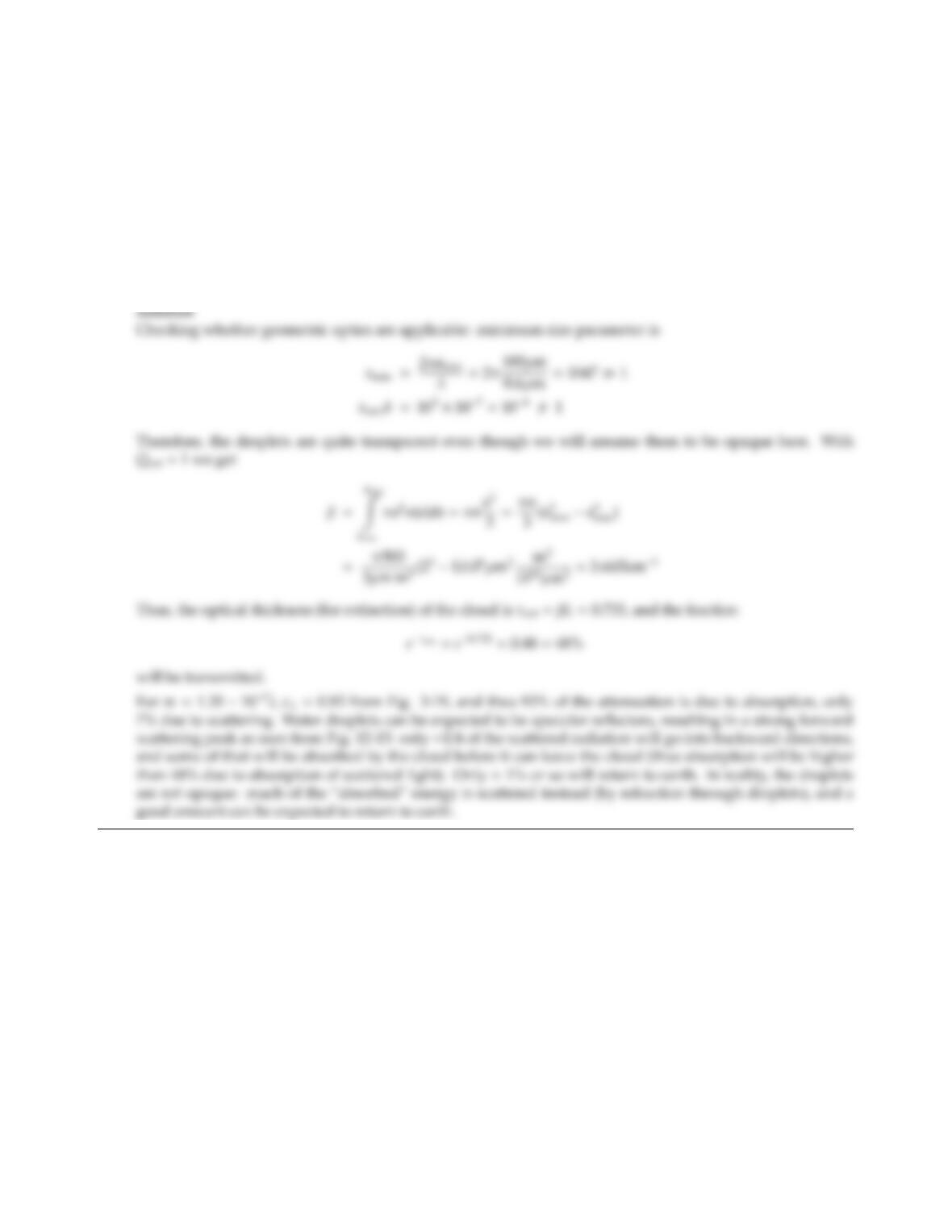12.10 A LIDAR laser beam (operating in the green at λ=0.6µm) is shot into the sky. At a height of 1km the laser
encounters a 200m thick cloud consisting of water droplets of varying size (100µma200µm), but constant
particle distribution function everywhere (n=500m m3).
What fraction of the laser beam will be transmitted through the cloud? How much will be absorbed? Very
approximately, how much would you expect to get scattered back to the earth’s surface? Carefully justify
your statements about absorption and scattering, using estimates, graphs and/or physical arguments for
support. Qualitatively, how would your explanation change, if you take into account that k=107(i.e.,
droplets are not opaque)?
Note: Water at 0.6µm has an index of refraction of m1.35 107i. For the sake of this problem you may
assume the droplets to be opaque (not really true).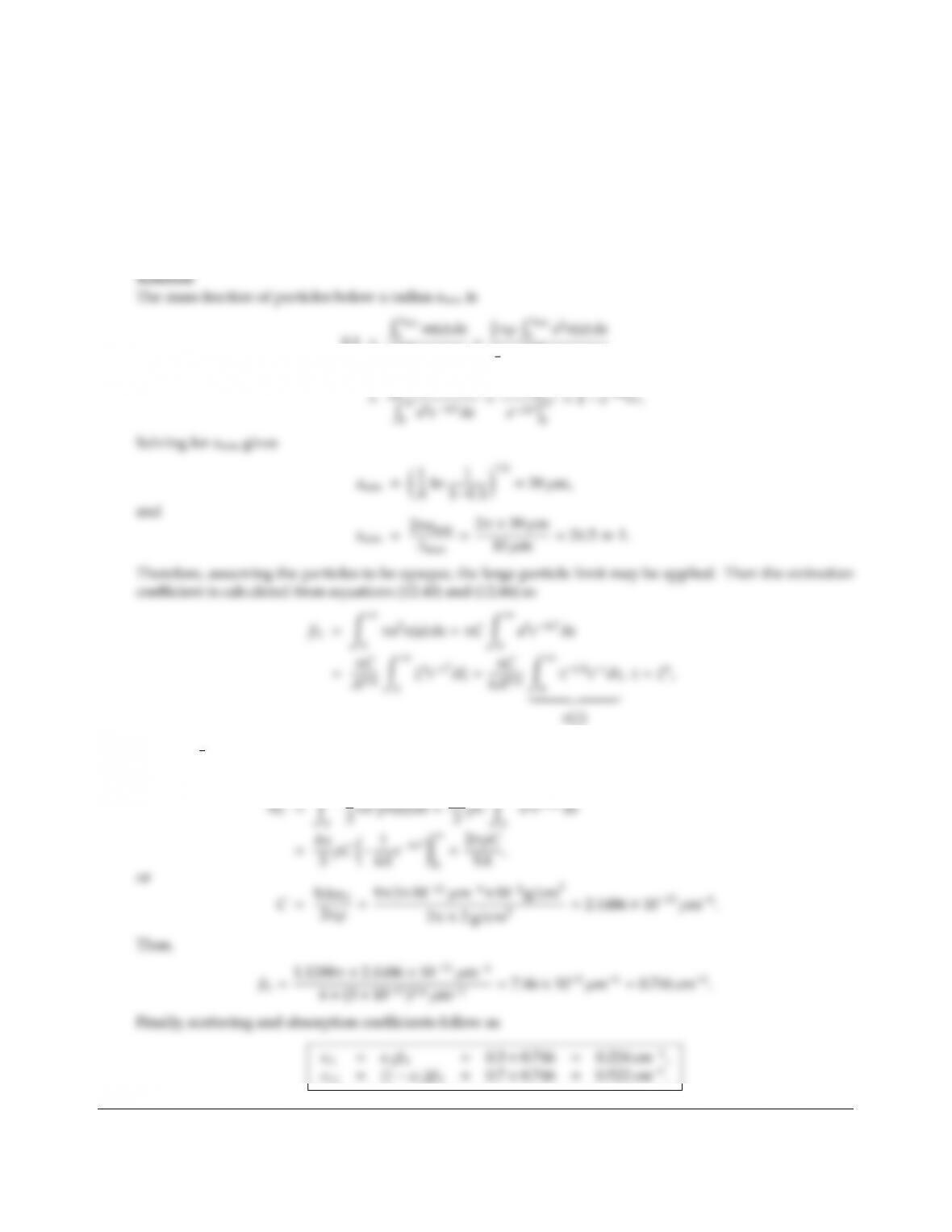CHAPTER 12 349
12.11 In a coal-burning plant, pulverized coal is used that is known to have a particle size distribution function of
n(a)a2eAa6,A=3×1011 µm6.
The coal may be approximated as diuse spheres with a gray emittance of ǫ=0.3. What is the eective
minimum size parameter, xmin, (i.e., 90% by weight of all particles have a size parameter larger than that)?
You may assume a combustion temperature of 2000 K, i.e., the relevant wavelengths range from about
1µm to about 10 µm. If the furnace is loaded with 10 kg coal particles per cubic meter, what are the spectral
absorption and scattering coecients? (Density of the coal =2000 kg/m3).
0.1=Ramin
R
0m(a)da
=
4
3πρ R
0a3n(a)da
0a5eAa6da
amin
0
where Γ5
6=1.1288 is the gamma function. The unknown constant Cis determined from the mass fraction,
which equals number of particles ×their individual masses, i.e.,
4

## Trusted by Thousands ofStudents

Here are what students say about us.